## General Question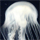# I have a vector physics problem, its for tonights homework so could some one help me A.S.A.P?

Asked by jdogg (871) March 1st, 2010

Okay, so the problem is: A boat is traveling in the ocean, 40 Newtons @ 45° North of East, the wind is blowing against it 75 Newtons @ 75° South of East, the current is moving 120 newtons due West. How many newtons and what direction and degrees is the boat moving… thanks :)

Observing members: 0Composing members: 0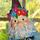Read your book. It tells you how to solve this kind of problem.

What part are you confused about? Sounds pretty straight forward to me.

lilikoi (10094)“Great Answer” (0) Flag as…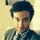What, specifically, are you having trouble with?

andrew (16380)“Great Answer” (0) Flag as…I just picked up the Vector physics for dummies book, be right with you in like ten minutes…

Ltryptophan (11049)“Great Answer” (1) Flag as…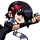Treat each force as a vector, breaking them each into their x (east-west) and y (north-south) coordinates. Then sum each vector by adding up the x and y components. Once you have that, find the magnitude of the resultant vector and use the inverse tangent function to find the angle from the x-axis.

Ivan (13479)“Great Answer” (4) Flag as…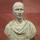Draw a diagram to show the direction and magnitude of each force. From there it is simple vector addition.

We are not here to do your homework for you – if you don’t understand it, your teacher will be able to explain it perfectly well. There is no point to pretending you can do something you can’t.

@Ivan There’s no point in breaking it into x and y components for this, adding head to tail with trig is adequate.

FireMadeFlesh (16563)“Great Answer” (2) Flag as…Either way works equally well, I just happen to like component form better.

Ivan (13479)“Great Answer” (1) Flag as…@Ivan Fair enough. I just don’t like adding extra steps.

FireMadeFlesh (16563)“Great Answer” (1) Flag as…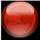Take each vector and take its magnitude (amount of Newtons) and then multiply the cosine of its angle (ex: cos(45)) This is the X component. Then take its magnitude and multiply by the sine of its angle. This is the Y component. Do this for each of the 3 forces and then simply add up the X and Y components. Now square each number (X and Y component) and add them up, then take the square root. This is the final magnitude (amount of newtons the boat is actually moving) Then take the inverse tangent of the Y value over the X value (tan^-1(Y/x)) and this is the angle the boat is actually traveling.

For everyone who is telling him not to ask homework questions on here, just don’t answer if it bothers you. Also, I don’t even think fluther generally has a problem with homework questions.

jackm (6207)“Great Answer” (1) Flag as…thank you for the people who answered, and by the way, I don’t want people to do it for me, I just need help grasping the new concept, luckily since someone who did try to help answer my question, I youtubed head-to-tail method, and breaking down x and y components and I understand it now, so more or less I got what I needed, and I want to let the people know who helped me thanks for their time!

jdogg (871)“Great Answer” (2) Flag as…or